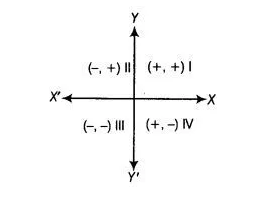# Point (-3, 5) lies in the`
Question:

Point (-3, 5) lies in the

Thinking Process

(i) Firstly, check the sign of each coordinate of a point.

(ii) If both coordinates x and y has same positive sign i.e., (+, +), then the point lies in first quadrant.

(iii) If x-coordinate has negative sign and y-coordinate has positive sign i.e., (-, +), then the point lies in second quadrant.

(iv) If both coordinate x and y has negative sing i.e, (-, -), then the point lies in third quadrant.

(v) If x-coordinate has positive sign and y-coordinate has negative sign i.e., (+, -), then the point lies in fourth quadrant

Solution:

(b) In point (-3, 5), x-coordinate is negative and y-coordinate is positive. So, the point lies in the second quadrant.